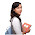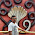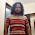Vedic Maths Shortcuts for Addition

Friends, we proudly introducing the lessons on Vedic Mathematics. Mrs. Rupali Shete is going to post articles on Easy Methods in Maths and Vedic Algebra (one lesson per day). We hope you will find these helpful, All the Best.  Today we shall discuss a basic vedic math shortcut method for finding additions.Adding two or more numbers is known as addition. We call it as sankalana in Sanskrit. Addition is the most easiest of all the operations in Mathematics. However, students as well as elders may get confusion while doing addition of large numbers, jumbled figures and digits not arranged according to place values. In rare cases even teachers get confusion in our traditional (presently following) method. So, to avoid such a situation, there is a method in 'Vedic Mathematics' known as "Bindvankana Method' which is very much useful, easier, practicable and advisable in the operation of additions. It is also called as 'Dot Method' which is clearly explained in the following example.

Example 1 : 759+587+998 = ?

Solution :
• Step 1 : As per our Traditional procedure, in the first step we should arrange them in a specific order such that they are in some specific rows and columns as per the place values of each number. Here, O, T and H represent Ones, Tens and Hundreds places respectively.

• Step 2 : Generally, while doing additions we add the digits in columns starting from right side i.e., from units place. In this method also we do the same but the adoption of procedure is a bit different.
• First Column :
• In the present example the first column consists of 8, 7 and 9 from bottom to top. Then, 8+7 is 15, we can take it as 10+5. Now, as a count of 10 (just like in our ancient abacus) keep a dot on 7 so that our procedure gets completed up to the digit 7.  (Here the point is, upto 10 we've completed one ten and the remainder is 5).
• Then the remaining 5 should be kept in our memory. Proceed in the same way with 5 and so that we get 14 . Again crossed 10, so we have to keep one dot on 9 (to represent the 10) and the remaining 4 we should take down to the answer row. The two dots on 7 and 9 are to be taken as 2 (if the number of dots are 3 then you should take 3)  and the same procedure is to be followed for the second column.
• Second Column :
• In the second column, we should add the 2 (taken from the first column) to 9, its 11 so crossed 10. Put dot mark at 9 and remainder 1. 8+1 is 9 (not reached 10 so move upwards). 5+9 = 14, so put dot at 5 and put the remainder 4 at the answer row. Now again we have got 2 dots, so take 2 to the next column.
• the same way we can continue the procedure irrespective of the number of rows and columns in the problem
• Third Column :
• Add 2 to 9 (9+2=11) it crosses 10, so put a dot mark at 9 and move upwards with the 1 in mind. 5+1=6 (not reached 10) so move upwards. 6+7=13 (crossed 10) so put dot mark on 7 and take that remainder 3 to the down. Now again we have got 2 dots. But there is no other column remaining at the left side so put that to in the answer row. That's all.
Important Points to note :
• Bindvankana Method is useful in addition with carrying digits.
• In Bindvankana Method of Addition we have to keep a dot on the respective digit after crossing 10 and continue addition with the remaining digit in mind.
• We have to count the number of dots and carry that number to the next column.
Now lets do some Exercises (Answers will be posted in tomorrow's lesson)
1. 789+456+587
2. 657+876+609
3. 568+956+546
4. 759+576+447
5. 869+974+349
6. 379+609+947
7. 678+547+349
8. 968+856+349
9. 3428+2356+5642
10. 4508+7823+2376
11. 9205+4506+7452
12. 2964+4536+6094
13. 9241+3462+5643
14. 1426+5307+2341
15. 2431+7032+3216
16. 5632+6354+4208
17. 23145+67098+56743+34523+45806
18. 32415+97806+74635+54323+80654
19. 14326+90786+74563+42327+26581
20. 78602+53098+19607+32543+26759
Check answers from hereRupali Shete (M.Sc., M.Phil., Ph.D) is a gold medalist from Osmania University (Hyderabad), one of the working members of Rananujan Mathematics Academy and Institute of Scientific Research on Vedas (ISERVE), Currently working in University of Pune.

1.Lakshmi Narayana PoojyulaNovember 30, 2013 at 8:21 PM

Thank you madam. Good One. Waiting for the remaining lessons :)

2.Can you please explain it more elaborately. . . I could not get it ma'm. Thank you

1.Elaborated now Friend. Please check once. Is it ok now ?

3.Good Initiative.. God Bless

4.i can't understand mam..can u plz explain it more..plzzz

1.Hi ..
you can go to this link . . . here is a video by some person . . he is explaining well .. Me too cleared my concept from here because its difficult to explaing in written as it needs more elaboration . . what ma'm has said is 100 percent correct but is in brief.. . .http://www.youtube.com/watch?v=SfhcRzVZUEk
TC

2.thanq..friend

5.Good one.....

1.can u explain step-2

2.Elaborated step 2 Sneha.. Please check and say, is it ok now ?

6.I cnt understand

7.oops i also can t understand madam der is no clarity

1.Check now Ajay... Is it ok now ?

2.Perfect

Thanks

3.i love vedic maths, its very interesting, and deep